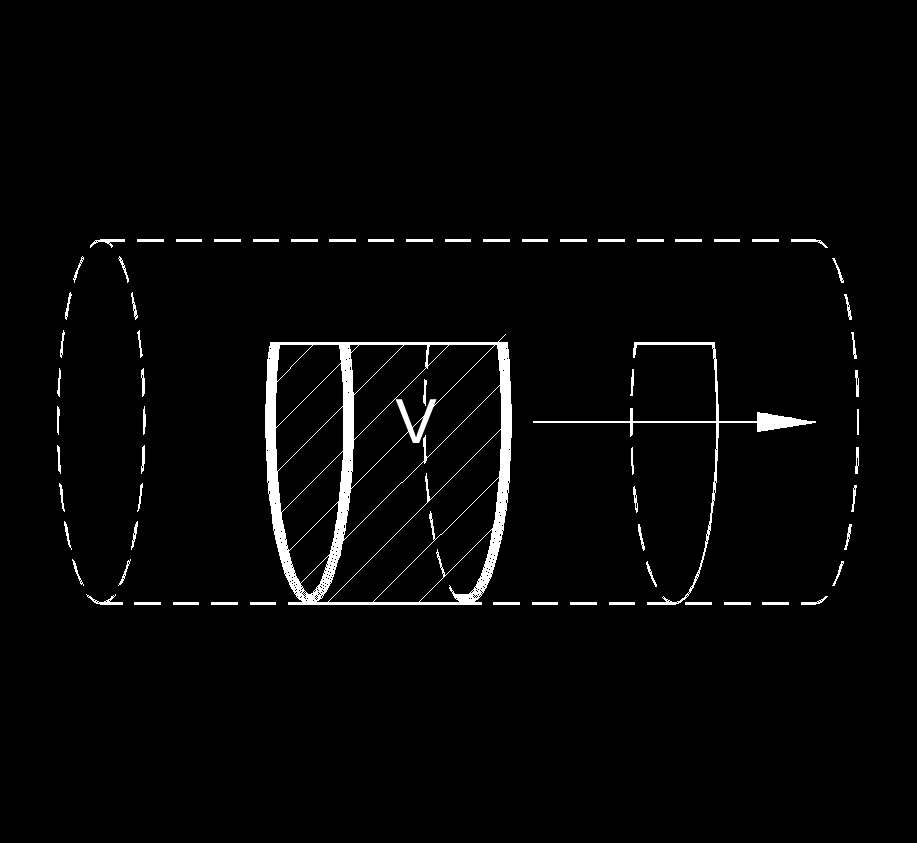Written by Jerry Ratzlaff on . Posted in Fluid DynamicsHydraulic gradient flow rate measure the amount of fluid that flows in a given time past a specific.

## Formulas that use Hydraulic Gradient Flow Rate

 $$\large{ Q = k \; i \; A_c }$$

### Where:

$$\large{ Q }$$ = flow rate

$$\large{ A_c }$$ = area cross-section of flow

$$\large{ k }$$ = hydraulic conductivity

$$\large{ i }$$ = hydraulic gradient